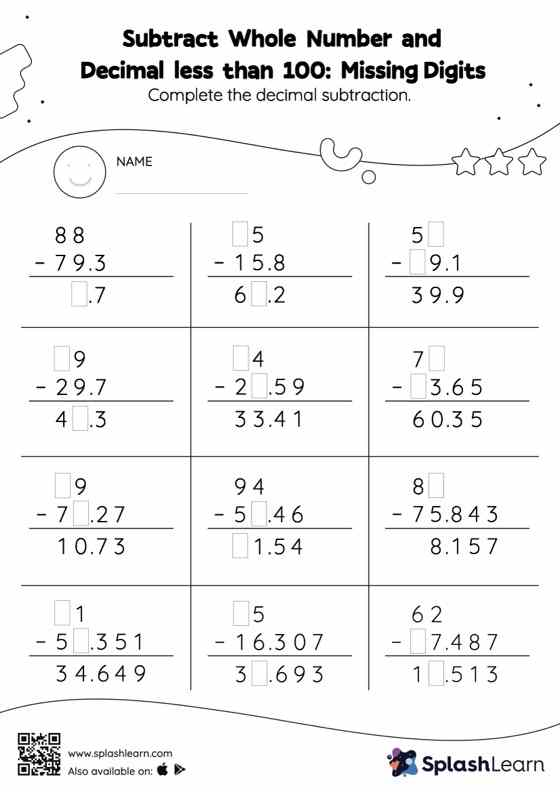# Subtract Whole Number and Decimal less than 100: Missing Digits Worksheet

Home > Subtract Whole Number and Decimal less than 100: Missing DigitsThis worksheet consists of a set of questions on subtraction where students are required to subtract whole number and decimal less than 100. In each problem, the numbers are laid out in the vertical format. In this format, pair of digits in each successive place are tackled one by one. This helps students follow a structured approach.